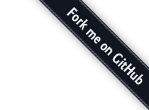## 1. 列表中的每项乘2

Marcus 的第一个例子演示了 map 函数，我们可以使用 range 语法以及一个匿名函数来完成同样的事情：

``````[1..10].map (i) -> i*2
``````

``````i * 2 for i in [1..10]
``````

## 2. 数列求和

Javascript（以及 CoffeeScript 扩展）同样有原生的 map 以及 reduce 函数：

``````    [1..1000].reduce (t, s) -> t + s

(reduce == reduceLeft, 还有reduceRight)
``````

## 3. 检查字符串是否包含某个单词

``````wordList = ["coffeescript", "eko", "play framework", "and stuff", "falsy"]
tweet = "This is an example tweet talking about javascript and stuff."

wordList.some (word) -> ~tweet.indexOf word
``````

``````wordList.filter (word) -> ~tweet.indexOf word
``````

`~` 在 CoffeeScript 中并不是什么特别的操作符，这里我们使用了一个小技巧，它实际上就是按位取反操作符， 会对数值按位进行取反操作，在上面的例子里它相当于 `-x-1`，这里我们用它来检查数组的下标是否大于 -1， 因为 `-(-1)-1 == 0`，会返回 `false`

## 4. 读取文件

``````fs.readFile 'data.txt', (err, data) -> fileText = data
``````

``````fileText = fs.readFileSync('data.txt').toString()
``````

## 5. 生日快乐

``````[1..4].map (i) -> console.log "Happy Birthday " + (if i is 3 then "dear Robert" else "to You")
``````

``````console.log "Happy Birthday #{if i is 3 then "dear Robert" else "to You"}" for i in [1..4]
``````

## 6. 过滤数列

``````passed = []
failed = []
(if score > 60 then passed else failed).push score for score in [49, 58, 76, 82, 88, 90]
``````

(也可以使用 `filter`，但是那样就不是一行了。。。）

## 7. 读取并解析一个XML Web service

XML是个神马东东？从没听过，让我们把它换成 json，你可以使用 request 库：

``````request.get { uri:'path/to/api.json', json: true }, (err, r, body) -> results = body
``````

## 8. 找到一个数列的最小（最大）值

``````Math.max.apply @, [14, 35, -7, 46, 98] # 98
Math.min.apply @, [14, 35, -7, 46, 98] # -7
``````

## 10. 埃拉托斯特尼筛法

（译者注：埃拉托斯特尼筛法是古希腊数学家埃拉托斯特尼所提出的一种简单的判定素数的算法，详细介绍请参见维基百科）

``````sieve = (num) ->
numbers = [2..num]
while ((pos = numbers) * pos) <= num
delete numbers[i] for n, i in numbers by pos
numbers.shift()
numbers.indexOf(num) > -1
``````

``````primes = []
primes.push i for i in [2..100] when not (j for j in primes when i % j == 0).length
``````

``````(n) -> (p.push i for i in [2..n] when not (j for j in (p or p=[]) when i%j == 0)) and n in p
``````

``````(n) -> (p.push i for i in [2..n] when !(p or p=[]).some((j) -> i%j is 0)) and n in p
``````

## 11. 奖励

``````"#{if i%3 is 0 then 'fizz' else ''}#{if i%5 is 0 then 'buzz' else ''}" or i for i in [1..100]
``````

``````['fizz' unless i%3] + ['buzz' unless i%5] or i for i in [1..100]
``````

## 结论